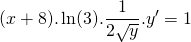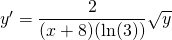# Solution assignment 09 Implicit differentiation

### Assignment 9

Calculate the tangent line at the pointof the graph of the following expression: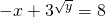### Solution

First we verify whether the given point is on the graph of the expression. This appears the case.
Next we calculate the derivative and do this by implicit differentiation.

The notation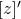means the derivative of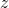.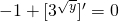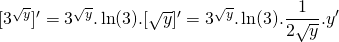We know that: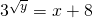and thus: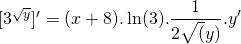From this we can derive: